# dplyr Tutorial : Data Manipulation (50 Examples)

The dplyr package is one of the most powerful and popular package in R. This package was written by the most popular R programmer Hadley Wickham who has written many useful R packages such as ggplot2, tidyr etc. This post includes several examples and tips of how to use dplyr package for cleaning and transforming data. It's a complete tutorial on data manipulation and data wrangling with R.

## What is dplyr?

The dplyr is a powerful R-package to manipulate, clean and summarize unstructured data. In short, it makes data exploration and data manipulation easy and fast in R.

The package "dplyr" comprises many functions that perform mostly used data manipulation operations such as applying filter, selecting specific columns, sorting data, adding or deleting columns and aggregating data. Another most important advantage of this package is that it's very easy to learn and use dplyr functions. Also easy to recall these functions. For example, filter() is used to filter rows.dplyr Tutorial
How to install and load dplyr package
To install the dplyr package, type the following command.
`install.packages("dplyr")`
To load dplyr package, type the command below
`library(dplyr)`

## Important dplyr Functions to remember

dplyr Function Description Equivalent SQL
select() Selecting columns (variables) SELECT
filter() Filter (subset) rows. WHERE
group_by() Group the data GROUP BY
summarise() Summarise (or aggregate) data -
arrange() Sort the data ORDER BY
join() Joining data frames (tables) JOIN
mutate() Creating New Variables COLUMN ALIAS

## dplyr vs. Base R Functions

dplyr functions process faster than base R functions. It is because dplyr functions were written in a computationally efficient manner. They are also more stable in the syntax and better supports data frames than vectors.
SQL Queries vs. dplyr
People have been utilizing SQL for analyzing data for decades. Every modern data analysis software such as Python, R, SAS etc supports SQL commands. But SQL was never designed to perform data analysis. It was rather designed for querying and managing data. There are many data analysis operations where SQL fails or makes simple things difficult. For example, calculating median for multiple variables, converting wide format data to long format etc. Whereas, dplyr package was designed to do data analysis.
`The names of dplyr functions are similar to SQL commands such as select() for selecting variables, group_by() - group data by grouping variable, join() - joining two data sets. Also includes inner_join() and left_join(). It also supports sub queries for which SQL was popular for.`
Data : Income Data by States
In this tutorial, we are using the following data which contains income generated by states from year 2002 to 2015. Note : This data do not contain actual income figures of the states. To download the dataset, click on this link - Dataset and then right click and hit Save as option.
This dataset contains 51 observations (rows) and 16 variables (columns). The snapshot of first 6 rows of the dataset is shown below.

```  Index      State   Y2002   Y2003   Y2004   Y2005   Y2006   Y2007   Y2008   Y2009
1     A    Alabama 1296530 1317711 1118631 1492583 1107408 1440134 1945229 1944173
2     A     Alaska 1170302 1960378 1818085 1447852 1861639 1465841 1551826 1436541
3     A    Arizona 1742027 1968140 1377583 1782199 1102568 1109382 1752886 1554330
4     A   Arkansas 1485531 1994927 1119299 1947979 1669191 1801213 1188104 1628980
5     C California 1685349 1675807 1889570 1480280 1735069 1812546 1487315 1663809
6     C   Colorado 1343824 1878473 1886149 1236697 1871471 1814218 1875146 1752387
Y2010   Y2011   Y2012   Y2013   Y2014   Y2015
1 1237582 1440756 1186741 1852841 1558906 1916661
2 1629616 1230866 1512804 1985302 1580394 1979143
3 1300521 1130709 1907284 1363279 1525866 1647724
4 1669295 1928238 1216675 1591896 1360959 1329341
5 1624509 1639670 1921845 1156536 1388461 1644607
6 1913275 1665877 1491604 1178355 1383978 1330736
```
Submit the following code to load data directly from link. If you want to load the data from your local drive, you need to change the file path in the code below.
```mydata = read.csv("https://raw.githubusercontent.com/deepanshu88/data/master/sampledata.csv")
```
dplyr Practical Examples
Example 1 : Selecting Random N Rows
The sample_n function selects random rows from a data frame (or table). The second parameter of the function tells R the number of rows to select.
`sample_n(mydata,3)`
```   Index    State   Y2002   Y2003   Y2004   Y2005   Y2006   Y2007   Y2008   Y2009
2      A   Alaska 1170302 1960378 1818085 1447852 1861639 1465841 1551826 1436541
8      D Delaware 1330403 1268673 1706751 1403759 1441351 1300836 1762096 1553585
33     N New York 1395149 1611371 1170675 1446810 1426941 1463171 1732098 1426216
Y2010   Y2011   Y2012   Y2013   Y2014   Y2015
2  1629616 1230866 1512804 1985302 1580394 1979143
8  1370984 1318669 1984027 1671279 1803169 1627508
33 1604531 1683687 1500089 1718837 1619033 1367705
```
Example 2 : Selecting Random Fraction of Rows
The sample_frac function returns randomly N% of rows. In the example below, it returns randomly 10% of rows.
`sample_frac(mydata,0.1)`
Example 3 : Remove Duplicate Rows based on all the variables (Complete Row)
The distinct function is used to eliminate duplicates.
`x1 = distinct(mydata)`
In this dataset, there is not a single duplicate row so it returned same number of rows as in mydata.
Example 4 : Remove Duplicate Rows based on a variable
The .keep_all function is used to retain all other variables in the output data frame.
`x2 = distinct(mydata, Index, .keep_all= TRUE)`
Example 5 : Remove Duplicates Rows based on multiple variables
In the example below, we are using two variables - Index, Y2010 to determine uniqueness.
`x2 = distinct(mydata, Index, Y2010, .keep_all= TRUE)`

## select( ) Function

It is used to select only desired variables.
```select() syntax : select(data , ....)
data : Data Frame
.... : Variables by name or by function```
Example 6 : Selecting Variables (or Columns)
Suppose you are asked to select only a few variables. The code below selects variables "Index", columns from "State" to "Y2008".
`mydata2 = select(mydata, Index, State:Y2008)`
Example 7 : Dropping Variables
The minus sign before a variable tells R to drop the variable.
`mydata = select(mydata, -Index, -State)`
The above code can also be written like :
`mydata = select(mydata, -c(Index,State))`
Example 8 : Selecting or Dropping Variables starts with 'Y'
The starts_with() function is used to select variables starts with an alphabet.
`mydata3 = select(mydata, starts_with("Y"))`
Adding a negative sign before starts_with() implies dropping the variables starts with 'Y'
`mydata33 = select(mydata, -starts_with("Y"))`
The following functions helps you to select variables based on their names.
Helpers Description
starts_with() Starts with a prefix
ends_with() Ends with a prefix
contains() Contains a literal string
matches() Matches a regular expression
num_range() Numerical range like x01, x02, x03.
one_of() Variables in character vector.
everything() All variables.
Example 9 : Selecting Variables contain 'I' in their names
`mydata4 = select(mydata, contains("I"))`
Example 10 : Reorder Variables
The code below keeps variable 'State' in the front and the remaining variables follow that.
`mydata5 = select(mydata, State, everything())`
New order of variables are displayed below -
```  "State" "Index" "Y2002" "Y2003" "Y2004" "Y2005" "Y2006" "Y2007" "Y2008" "Y2009"
 "Y2010" "Y2011" "Y2012" "Y2013" "Y2014" "Y2015"```

## rename( ) Function

It is used to change variable name.
```rename() syntax : rename(data , new_name = old_name)
data : Data Frame
new_name : New variable name you want to keep
old_name : Existing Variable Name```
Example 11 : Rename Variables
The rename function can be used to rename variables.

In the following code, we are renaming 'Index' variable to 'Index1'.
`mydata6 = rename(mydata, Index1=Index)`Output

## filter( ) Function

It is used to subset data with matching logical conditions.
```filter() syntax : filter(data , ....)
data : Data Frame
.... : Logical Condition```
Example 12 : Filter Rows
Suppose you need to subset data. You want to filter rows and retain only those values in which Index is equal to A.
`mydata7 = filter(mydata, Index == "A")`
``` Index    State   Y2002   Y2003   Y2004   Y2005   Y2006   Y2007   Y2008   Y2009
1     A  Alabama 1296530 1317711 1118631 1492583 1107408 1440134 1945229 1944173
2     A   Alaska 1170302 1960378 1818085 1447852 1861639 1465841 1551826 1436541
3     A  Arizona 1742027 1968140 1377583 1782199 1102568 1109382 1752886 1554330
4     A Arkansas 1485531 1994927 1119299 1947979 1669191 1801213 1188104 1628980
Y2010   Y2011   Y2012   Y2013   Y2014   Y2015
1 1237582 1440756 1186741 1852841 1558906 1916661
2 1629616 1230866 1512804 1985302 1580394 1979143
3 1300521 1130709 1907284 1363279 1525866 1647724
4 1669295 1928238 1216675 1591896 1360959 1329341
```
Example 13 : Multiple Selection Criteria
The %in% operator can be used to select multiple items. In the following program, we are telling R to select rows against 'A' and 'C' in column 'Index'.
`mydata7 = filter(mydata6, Index %in% c("A", "C"))`
Example 14 : 'AND' Condition in Selection Criteria
Suppose you need to apply 'AND' condition. In this case, we are picking data for 'A' and 'C' in the column 'Index' and income greater than 1.3 million in Year 2002.
`mydata8 = filter(mydata6, Index %in% c("A", "C") & Y2002 >= 1300000 )`
Example 15 : 'OR' Condition in Selection Criteria
The 'I' denotes OR in the logical condition. It means any of the two conditions.
`mydata9 = filter(mydata6, Index %in% c("A", "C") | Y2002 >= 1300000)`
Example 16 : NOT Condition
The "!" sign is used to reverse the logical condition.
`mydata10 = filter(mydata6, !Index %in% c("A", "C"))`
Example 17 : CONTAINS Condition
The grepl function is used to search for pattern matching. In the following code, we are looking for records wherein column state contains 'Ar' in their name.
`mydata10 = filter(mydata6, grepl("Ar", State))`

## summarise( ) Function

It is used to summarize data.
```summarise() syntax : summarise(data , ....)
data : Data Frame
..... : Summary Functions such as mean, median etc```
Example 18 : Summarize selected variables
In the example below, we are calculating mean and median for the variable Y2015.
`summarise(mydata, Y2015_mean = mean(Y2015), Y2015_med=median(Y2015))`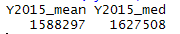Output
Example 19 : Summarize Multiple Variables
In the following example, we are calculating number of records, mean and median for variables Y2005 and Y2006. The summarise_at function allows us to select multiple variables by their names.
```summarise_at(mydata, vars(Y2005, Y2006), funs(n(), mean, median))
```
`funs( )` has been soft-deprecated (dropped) from dplyr 0.8.0. Instead we should use `list`. The equivalent code is stated below -
```summarise_at(mydata, vars(Y2005, Y2006), list(n=~n(), mean=mean, median=median))
```
Another way of using it without stating names is through formula instead of function. This is `mean = mean` function and this is `~mean(.)` formula.
```summarise_at(mydata, vars(Y2005, Y2006), list(~n(), ~mean(.), ~median(.)))
```
You must be wondering about `~` and `.` symbols. It's a way to pass purrr style anonymous function. See the base R method as compared to purrr style below. Both returns the same output. purrr style provides a shortcut to define anonymous function.
Base R Style
```summarise_at(mydata, vars(Y2005, Y2006), function(x) length(unique(x)))
```
purrr Style
```summarise_at(mydata, vars(Y2005, Y2006), ~length(unique(.)))
```
Symbols `.` and `.x` means the same thing. You can try the above code by replacing `.` with `.x`Output
Example 20 : Summarize with Custom Functions
Incase you want to add additional arguments for the functions mean and median (for example na.rm = TRUE), you can do it like the code below.
```summarise_at(mydata, vars(Y2011, Y2012),funs(mean, median), na.rm = TRUE)
```
We can also use custom functions in the summarise function. In this case, we are computing the number of records, number of missing values, mean and median for variables Y2011 and Y2012. The dot (.) denotes each variables specified in the second argument of the function.
```summarise_at(mydata, vars(Y2011, Y2012),
funs(n(), missing = sum(is.na(.)), mean(., na.rm = TRUE), median(.,na.rm = TRUE)))```
Instead of funs( ), you should make a habit of using list( ) as funs( ) can be dropped in future versions of dplyr package.
```summarise_at(mydata, vars(Y2011, Y2012),
list(~n(), missing = ~sum(is.na(.)), ~mean(., na.rm = TRUE),
~median(.,na.rm = TRUE)))
```Summarize : Output
How to apply Non-Standard Functions
Suppose you want to subtract mean from its original value and then calculate variance of it.
```set.seed(222)
mydata <- data.frame(X1=sample(1:100,100), X2=runif(100))
summarise_at(mydata,vars(X1,X2), function(x) var(x - mean(x)))```
```    X1         X2
1 841.6667 0.08142161
```
Equivalent purrr style method can be written like this :
```summarise_at(mydata,vars(X1,X2), ~ var(. - mean(.)))
```
Example 21 : Summarize all Numeric Variables
The summarise_if function allows you to summarise conditionally.
`summarise_if(mydata, is.numeric, funs(n(),mean,median))`
Alternative Method :
First, store data for all the numeric variables
`numdata = mydata[sapply(mydata,is.numeric)]`
Second, the summarise_all function calculates summary statistics for all the columns in a data frame
`summarise_all(numdata, funs(n(),mean,median))`
Example 22 : Summarize Factor Variable
We are checking the number of levels/categories and count of missing observations in a categorical (factor) variable.
`summarise_all(mydata["Index"], funs(nlevels(.), nmiss=sum(is.na(.))))`
```  nlevels nmiss
1      19   0
```

## arrange() function

Use : Sort data
Syntax
```arrange(data_frame, variable(s)_to_sort)
or
data_frame %>% arrange(variable(s)_to_sort)```
To sort a variable in descending order, use desc(x).
Example 23 : Sort Data by Multiple Variables
The default sorting order of arrange() function is ascending. In this example, we are sorting data by multiple variables.
`arrange(mydata, Index, Y2011)`
Suppose you need to sort one variable by descending order and other variable by ascending oder.
`arrange(mydata, desc(Index), Y2011)`

## Pipe Operator %>%

It is important to understand the pipe (%>%) operator before knowing the other functions of dplyr package. dplyr utilizes pipe operator from another package (magrittr).
`It allows you to write sub-queries like we do it in sql.`
Note : All the functions in dplyr package can be used without the pipe operator. The question arises "Why to use pipe operator %>%". The answer is it lets to wrap multiple functions together with the use of %>%.
Syntax :
```filter(data_frame, variable == value)
or
data_frame %>% filter(variable == value)```
The %>% is NOT restricted to filter function. It can be used with any function.
Example :
The code below demonstrates the usage of pipe %>% operator. In this example, we are selecting 10 random observations of two variables "Index" "State" from the data frame "mydata".
```dt = sample_n(select(mydata, Index, State),10)
or
dt = mydata %>% select(Index, State) %>% sample_n(10)```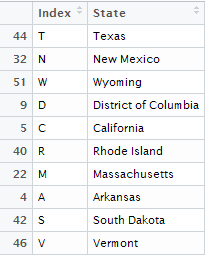Output

## group_by() function

Use : Group data by categorical variable
Syntax :
```group_by(data, variables)
or
data %>% group_by(variables)```
Example 24 : Summarise Data by Categorical Variable
We are calculating count and mean of variables Y2011 and Y2012 by variable Index.
`t = summarise_at(group_by(mydata, Index), vars(Y2011, Y2012), funs(n(), mean(., na.rm = TRUE)))`
The above code can also be written like
```t = mydata %>% group_by(Index) %>%
summarise_at(vars(Y2011:Y2015), funs(n(), mean(., na.rm = TRUE)))```
```Index Y2011_n Y2012_n  Y2013_n Y2014_n Y2015_n Y2011_mean Y2012_mean
A 4 4 4 4 4 1432642 1455876
C 3 3 3 3 3 1750357 1547326
D 2 2 2 2 2 1336059 1981868
F 1 1 1 1 1 1497051 1131928
G 1 1 1 1 1 1851245 1850111
H 1 1 1 1 1 1902816 1695126
I 4 4 4 4 4 1690171 1687056
K 2 2 2 2 2 1489353 1899773
L 1 1 1 1 1 1210385 1234234
M 8 8 8 8 8 1582714 1586091
N 8 8 8 8 8 1448351 1470316
O 3 3 3 3 3 1882111 1602463
P 1 1 1 1 1 1483292 1290329
R 1 1 1 1 1 1781016 1909119
S 2 2 2 2 2 1381724 1671744
T 2 2 2 2 2 1724080 1865787
U 1 1 1 1 1 1288285 1108281
V 2 2 2 2 2 1482143 1488651
W 4 4 4 4 4 1711341 1660192
```
Since dplyr >= 1.0.0 version you may get the following warnings.
```#`summarise()` ungrouping output (override with `.groups` argument)
#`summarise()` regrouping output by xxx (override with `.groups` argument)
```
To suppress this warning you can use the following command.
options(dplyr.summarise.inform=F)

## do() function

Use : Compute within groups
Syntax :
`do(data_frame, expressions_to_apply_to_each_group)`
Note : The dot (.) is required to refer to a data frame.
Example 25 : Filter Data within a Categorical Variable
Suppose you need to pull top 2 rows from 'A', 'C' and 'I' categories of variable Index.
```t = mydata %>% filter(Index %in% c("A", "C","I")) %>% group_by(Index) %>%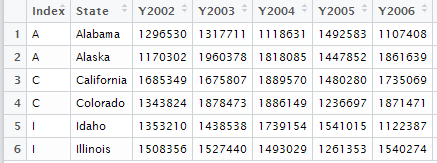Output : do() function
Example 26 : Selecting 3rd Maximum Value by Categorical Variable
We are calculating third maximum value of variable Y2015 by variable Index. The following code first selects only two variables Index and Y2015. Then it filters the variable Index with 'A', 'C' and 'I' and then it groups the same variable and sorts the variable Y2015 in descending order. At last, it selects the third row.
```t = mydata %>% select(Index, Y2015) %>%
filter(Index %in% c("A", "C","I")) %>%
group_by(Index) %>%
do(arrange(.,desc(Y2015))) %>%  slice(3)```
The slice() function is used to select rows by position.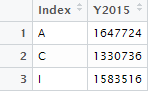Output
Using Window Functions
Like SQL, dplyr uses window functions that are used to subset data within a group. It returns a vector of values. We could use min_rank() function that calculates rank in the preceding example,
```t = mydata %>% select(Index, Y2015) %>%
filter(Index %in% c("A", "C","I")) %>%
group_by(Index) %>%
filter(min_rank(desc(Y2015)) == 3)```
```  Index   Y2015
1    A  1647724
2    C  1330736
3    I  1583516
```
Example 27 : Summarize, Group and Sort Together
In this case, we are computing mean of variables Y2014 and Y2015 by variable Index. Then sort the result by calculated mean variable Y2015.
```t = mydata %>%
group_by(Index)%>%
summarise(Mean_2014 = mean(Y2014, na.rm=TRUE),
Mean_2015 = mean(Y2015, na.rm=TRUE)) %>%
arrange(desc(Mean_2015))```

## mutate() function

Use :Creates new variables
Syntax :
```mutate(data_frame, expression(s) )
or
data_frame %>% mutate(expression(s))                ```
Example 28 : Create a new variable
The following code calculates division of Y2015 by Y2014 and name it "change".
`mydata1 = mutate(mydata, change=Y2015/Y2014)`
Example 29 : Multiply all the variables by 1000
It creates new variables and name them with suffix "_new".
`mydata11 = mutate_all(mydata, funs("new" = .* 1000))`Output
The output shown in the image above is truncated due to high number of variables.

Note - The above code returns the following error messages -

Warning messages:
1: In Ops.factor(c(1L, 1L, 1L, 1L, 2L, 2L, 2L, 3L, 3L, 4L, 5L, 6L, :
‘*’ not meaningful for factors
2: In Ops.factor(1:51, 1000) : ‘*’ not meaningful for factors

It implies you are multiplying 1000 to string(character) values which are stored as factor variables. These variables are 'Index', 'State'. It does not make sense to apply multiplication operation on character variables. For these two variables, it creates newly created variables which contain only NA.

Solution : See Example 45 - Apply multiplication on only numeric variables
Example 30 : Calculate Rank for Variables
Suppose you need to calculate rank for variables Y2008 to Y2010.
`mydata12 = mutate_at(mydata, vars(Y2008:Y2010), funs(Rank=min_rank(.)))`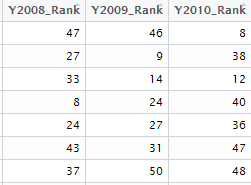Output
By default, min_rank() assigns 1 to the smallest value and high number to the largest value. In case, you need to assign rank 1 to the largest value of a variable, use min_rank(desc(.))
`mydata13 = mutate_at(mydata, vars(Y2008:Y2010), funs(Rank=min_rank(desc(.))))`
Example 31 : Select State that generated highest income among the variable 'Index'
```out = mydata %>% group_by(Index) %>% filter(min_rank(desc(Y2015)) == 1) %>%
select(Index, State, Y2015)```
```    Index         State   Y2015
2      C   Connecticut 1718072
3      D      Delaware 1627508
4      F       Florida 1170389
5      G       Georgia 1725470
6      H        Hawaii 1150882
7      I         Idaho 1757171
8      K      Kentucky 1913350
9      L     Louisiana 1403857
10      M      Missouri 1996005
11      N New Hampshire 1963313
12      O        Oregon 1893515
13      P  Pennsylvania 1668232
14      R  Rhode Island 1611730
15      S  South Dakota 1136443
16      T         Texas 1705322
17      U          Utah 1729273
18      V      Virginia 1850394
19      W       Wyoming 1853858
```
Example 32 : Cumulative Income of 'Index' variable
The cumsum function calculates cumulative sum of a variable. With mutate function, we insert a new variable called 'Total' which contains values of cumulative income of variable Index.
```out2 = mydata %>% group_by(Index) %>% mutate(Total=cumsum(Y2015)) %>%
select(Index, Y2015, Total)```

## join() function

Use : Join two datasets
Syntax :
```inner_join(x, y, by = )
left_join(x, y, by = )
right_join(x, y, by = )
full_join(x, y, by = )
semi_join(x, y, by = )
anti_join(x, y, by = )```
x, y - datasets (or tables) to merge / join
by - common variable (primary key) to join by.
Example 33 : Common rows in both the tables
```df1 = data.frame(ID = c(1, 2, 3, 4, 5),
w = c('a', 'b', 'c', 'd', 'e'),
x = c(1, 1, 0, 0, 1),
y=rnorm(5),
z=letters[1:5])
df2 = data.frame(ID = c(1, 7, 3, 6, 8),
a = c('z', 'b', 'k', 'd', 'l'),
b = c(1, 2, 3, 0, 4),
c =rnorm(5),
d =letters[2:6])
```
INNER JOIN returns rows when there is a match in both tables. In this example, we are merging df1 and df2 with ID as common variable (primary key).
`df3 = inner_join(df1, df2, by = "ID")`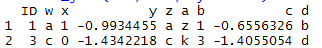Output : INNER JOIN
If the primary key does not have same name in both the tables, try the following way:
`inner_join(df1, df2, by = c("ID"="ID1"))`
Example 34 : Applying LEFT JOIN
LEFT JOIN : It returns all rows from the left table, even if there are no matches in the right table.
`left_join(df1, df2, by = "ID")`Output : LEFT JOIN

## Combine Data Vertically

intersect(x, y)
Rows that appear in both x and y.
union(x, y)
Rows that appear in either or both x and y.
setdiff(x, y)
Rows that appear in x but not y.
Example 35 : Applying INTERSECT
Prepare Sample Data for Demonstration
```mtcars\$model <- rownames(mtcars)
first <- mtcars[1:20, ]
second <- mtcars[10:32, ]```
INTERSECT selects unique rows that are common to both the data frames.
`intersect(first, second)`
Example 36 : Applying UNION
UNION displays all rows from both the tables and removes duplicate records from the combined dataset. By using union_all function, it allows duplicate rows in the combined dataset.
```x=data.frame(ID = 1:6, ID1= 1:6)
y=data.frame(ID = 1:6,  ID1 = 1:6)
union(x,y)
union_all(x,y)```
Example 37 : Rows appear in one table but not in other table
`setdiff(first, second)`
Example 38 : IF ELSE Statement
Syntax :
`if_else(condition, true, false, missing = NULL)`
true : Value if condition meets
false : Value if condition does not meet
missing : Value if missing cases.It will be used to replace missing values (Default : NULL)
```df <- c(-10,2, NA)
if_else(df < 0, "negative", "positive", missing = "missing value")```
Create a new variable with IF_ELSE
If a value is less than 5, add it to 1 and if it is greater than or equal to 5, add it to 2. Otherwise 0.
```df =data.frame(x = c(1,5,6,NA))
df %>% mutate(newvar=if_else(x<5, x+1, x+2,0))```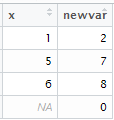Output
Nested IF ELSE
Multiple IF ELSE statement can be written using if_else() function. See the example below -
```mydf =data.frame(x = c(1:5,NA))
mydf %>% mutate(newvar= if_else(is.na(x),"I am missing",
if_else(x==1,"I am one",
if_else(x==2,"I am two",
if_else(x==3,"I am three","Others")))))```
Output
```   x         flag
1  1     I am one
2  2     I am two
3  3   I am three
4  4       Others
5  5       Others
6 NA I am missing
```
SQL-Style CASE WHEN Statement
We can use case_when() function to write nested if-else queries. In case_when(), you can use variables directly within case_when() wrapper. TRUE refers to ELSE statement.
```mydf %>% mutate(flag = case_when(is.na(x) ~ "I am missing",
x == 1 ~ "I am one",
x == 2 ~ "I am two",
x == 3 ~ "I am three",
TRUE ~ "Others"))
```
Important Point
`Make sure you set is.na() condition at the beginning in nested ifelse. Otherwise, it would not be executed.`
Example 39 : Apply ROW WISE Operation
Suppose you want to find maximum value in each row of variables 2012, 2013, 2014, 2015. The rowwise() function allows you to apply functions to rows.
```df = mydata %>%
rowwise() %>% mutate(Max= max(Y2012,Y2013,Y2014,Y2015)) %>%
select(Y2012:Y2015,Max)```
Example 40 : Combine Data Frames
Suppose you are asked to combine two data frames. Let's first create two sample datasets.
```df1=data.frame(ID = 1:6,  x=letters[1:6])
df2=data.frame(ID = 7:12, x=letters[7:12])```Input Datasets
The bind_rows() function combine two datasets with rows. So combined dataset would contain 12 rows (6+6) and 2 columns.
`xy = bind_rows(df1,df2)`
It is equivalent to base R function rbind.
`xy = rbind(df1,df2)`
The bind_cols() function combine two datasets with columns. So combined dataset would contain 4 columns and 6 rows.
```xy = bind_cols(x,y)
or
xy = cbind(x,y)```
The output is shown below-cbind Output
Example 41 : Calculate Percentile Values
The quantile() function is used to determine Nth percentile value. In this example, we are computing percentile values by variable Index.
```mydata %>% group_by(Index) %>%
summarise(Pecentile_25=quantile(Y2015, probs=0.25),
Pecentile_50=quantile(Y2015, probs=0.5),
Pecentile_75=quantile(Y2015, probs=0.75),
Pecentile_99=quantile(Y2015, probs=0.99))
```
The ntile() function is used to divide the data into N bins.
```x= data.frame(N= 1:10)
x = mutate(x, pos = ntile(x\$N,5))```
Example 42 : Automate Model Building
This example explains the advanced usage of do() function. In this example, we are building linear regression model for each level of a categorical variable. There are 3 levels in variable cyl of dataset mtcars.
`length(unique(mtcars\$cyl))`
Result : 3
```by_cyl <- group_by(mtcars, cyl)
models <- by_cyl %>% do(mod = lm(mpg ~ disp, data = .))
summarise(models, rsq = summary(mod)\$r.squared)
models %>% do(data.frame(
var = names(coef(.\$mod)),
coef(summary(.\$mod)))
)
```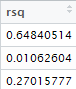Output : R-Squared Values

## if() Family of Functions

It includes functions like select_if, mutate_if, summarise_if. They come into action only when logical condition meets. See examples below.
Example 43 : Select only numeric columns
The select_if() function returns only those columns where logical condition is TRUE. The is.numeric refers to retain only numeric variables.
`mydata2 = select_if(mydata, is.numeric)`
Similarly, you can use the following code for selecting factor columns -
`mydata3 = select_if(mydata, is.factor)`
Example 44 : Number of levels in factor variables
Like select_if() function, summarise_if() function lets you to summarise only for variables where logical condition holds.
`summarise_if(mydata, is.factor, funs(nlevels(.)))`
It returns 19 levels for variable Index and 51 levels for variable State.
Example 45 : Multiply by 1000 to numeric variables
`mydata11 = mutate_if(mydata, is.numeric, funs("new" = .* 1000))`
Example 46 : Convert value to NA
In this example, we are converting "" to NA using na_if() function.
```k <- c("a", "b", "", "d")
na_if(k, "")```
Result : "a" "b" NA "d"
Example 47 : Use of pull( ) function
`iris %>% pull(Sepal.Length)` is equivalent to writing `iris\$Sepal.Length` or `iris[["Sepal.Length"]]` If you want output to be in vector rather than data frame (default method), you can use pull( ) function.
```iris %>% filter(Sepal.Length > 5.5) %>% pull(Species)
```
Example 48 : How to deal with Quotation
Let's understand with example. You want to use a variable which is in quotes. In the example below, Species is in quotes. If you use quoted variable directly, it would return zero rows. To make it work, you need to use `!!` operator which unquotes its argument and gets evaluated immediately in the surrounding context. The final thing we need to do is turn the character string "Species" into Species, a symbol by using `sym` function.
```filter_df <- function(df, colname, val){
filter(df, colname == val)
}
filter_df(iris,"Species", "setosa")

Output
Zero rows
```
```filter_df <- function(df, colname, val){
filter(df, !!sym(colname) == val)
}

filter_df(iris,"Species", "setosa")

Output
50 rows
```

`enquo()` is used to quote its argument. Here we are asking user to define variable name without quotes.

```filter_df <- function(df, colname, val){
colname = enquo(colname)
filter(df, !!colname == val)
}
filter_df(iris, Species, "setosa")
```

Example 49 : How to use SQL rank() over(partition by)
In SQL, `rank() over(partition by)` is used to compute rank by a grouping variable. In dplyr, it can be achieved very easily with a single line of code. See the example below. Here we are calculating rank of variable Y2015 by variable Index.
```t = mydata %>% select(Index, Y2015) %>%
group_by(Index) %>%
mutate(rank = min_rank(desc(Y2015)))%>%
arrange(Index, rank)
```
In dplyr, there are many functions to compute rank other than `min_rank( )`. These are `dense_rank( )`, `row_number( )`, `percent_rank()`.

## across() function

across( ) function was added starting dplyr version 1.0. It helps analyst to perform same operation on multiple columns. Let's take a sample data.frame `mtcars` and calculate mean on variables from 'mpg' through 'qsec' by 'carb'.
Alternative to `summarise_at` function
```mtcars %>%
group_by(carb) %>%
summarise(across(mpg:qsec, mean))
```
Alternative to `summarise_if` function
The code below calculates average on numeric variables. It identifies numeric variables using where() function.
```  mtcars %>%
group_by(carb) %>%
summarise(across(where(is.numeric), mean))
```
Multiple `across()` function
Here we are using two summary statistics - mean and no. of distinct values in two different set of variables.
```  mtcars %>%
group_by(carb) %>%
summarise(across(mpg:qsec, mean), across(vs:gear, n_distinct))
```
`across()` can also be applied with mutate function
```  mtcars %>%
group_by(carb) %>%
mutate(across(where(is.numeric), mean))
```
Some other examples of across() function -
df %>% mutate(across(c(x, starts_with("y")), mean, na.rm = TRUE))
df %>% mutate(across(everything(), mean, na.rm = TRUE))
Endnotes
There are hundreds of packages that are dependent on this package. The main benefit it offers is to take off fear of R programming and make coding effortless and lower processing time. However, some R programmers prefer data.table package for its speed. I would recommend learn both the packages. The data.table package wins over dplyr in terms of speed if data size greater than 1 GB.Share
Related PostsDeepanshu founded ListenData with a simple objective - Make analytics easy to understand and follow. He has over 10 years of experience in data science. During his tenure, he has worked with global clients in various domains like Banking, Insurance, Private Equity, Telecom and Human Resource.

51 Responses to "dplyr Tutorial : Data Manipulation (50 Examples)"
1.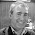Thanks for share, great stuff and examples.

1.Thank you for stopping by my blog!

2.This is the best tutorial out there

3.excellent!

thx

Z

4.Thank you, this is very helpful.

1.5.Having searched many sites and lectures I am bookmarking your site after looking at this page. Its the simplicity of your presentation. Thanks.

1.Thank you for stopping by my blog. Glad you found it useful. Cheers!

6.Thank you, this indeed very helpful and precise. Great Job!

1.7.I followed along your script step by step and got a warning message
in Example 29 : Multiply all the variables by 1000 as follows:
1: In Ops.factor(c(1L, 1L, 1L, 1L, 2L, 2L, 2L, 3L, 3L, 4L, 5L, 6L, :
‘*’ not meaningful for factors
2: In Ops.factor(1:51, 1000) : ‘*’ not meaningful for factors
What did it mean? Could you please give me some explanation. Thanks.

1.This error says 'multiplying 1000 on factor(string) variables' does not make sense. Run this command - str(mydata[,1:2])
First two variables in the dataframe mydata are strings that are stored as factor variables.

2.I got it. Thanks. I think I'm ready to go next to your another tutorial - data.table. It's quite interesting to learn R from your blog posts.

8.Example#22 - gives incorrect number of levels as 0 - fix is below
Why doesn't nlevels() work?

> summarise_all(dt["Index"], funs(nlevels(.), sum(is.na(.))))
# A tibble: 1 × 2
nlevels sum

1 0 0
===============
The fix is change nlevels() to length(unique(.)) as below

summarise_all(dt["Index"], funs(length(unique(.)), sum(is.na(.))))
# A tibble: 1 × 2
length sum

1 19 0

1.It works fine at my end. Check out the code below -
library(dplyr)
summarise_all(mydata["Index"], funs(nlevels(.), sum(is.na(.))))

9.Example #21

Alternatively, we can use the following:

mydata %>% summarise_if(is.numeric, funs(n(),mean,median))

1.Thank you for posting alternative method. I have added it to the tutorial. Cheers!

10.11.This is a great tutorial. A doubt that crept to me when I tries to mix multiple functions. Any reason why the following is not working:

DF <- mutate_if(mydata, is.numeric & contains('Y2015'), funs('new' = *.100));

but this works:
DF <- mutate_if(mydata, is.numeric, funs('new' = *.100));

what if I want to mutate to add only a column for Y2015?

Thanks

12.Great tutorials. Took too much time to found this tutorial.

13.Wonderfull for a newcomer to R !

14.Thank you for this great presentation.

15.Example 39 is wrong.

1.Try to use below code instead.
df = mydata %>%
rowwise() %>% mutate(Max= max(Y2012,Y2013,Y2014,Y2015)) %>%
select(Y2012:Y2015,Max)

16.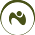17.W. r. t. chapter 'SQL-Style CASE WHEN Statement': The workaround .\$ is not necessary anymore from dplyr version 0.7.0

1.Updated. Thanks for pointing it out!

18.19.20.Data manipulation in R using data.table package tutorials is not available. Please fix that

1.Thanks for highlighting. It's fixed now!

21.Great tutorial.

22.Simply superb..Likes your blog a lot..CLEAR CUT EXPLANATION. Super

23.So many functions explained in such a simple and "easy-to-understand" manner.. Thanks a lot !! :)

1.24.Every example is precise, simple and very well explained. Many thanks and congrats!

25.love the detailed yet simple explanations.

26.mydata %>% filter(Index %in% c("A", "C","I")) %>% group_by(Index) %>%do(head( . , 2))

why am i getting different answers using these codes. Codes are same i guess

27.é de longe um dos melhores e mais completos tutorias sobre Dplyr. Obrigado!

28.I am Very thankful to you bro. Bec of u, i have learned dplyr and am using regularly.

29.sample_n function is not working

1.What error you are getting? Try this :
dplyr::sample_n(iris,3)

30.31.Really happy to come across this blog...helped me a lot!!

1.Thank you for stopping by my blog. Cheers!

32.Any code equivalent for over() (Partition By) function of SQL in DPLYR ?

1.I added example 49 for the same. Hope it helps!

33.Sir, powerful package for data manipulations and you made it very easy with your crystal clear explanations with examples.. very helpful stuff made me to learn in two days.. bookmarking your page. Thanks a lot and keep posting for R shiny if possible.. :)

34.Excellent tutorial , this has helped me a lot

35.Excellent tutorial and explanations are easy to understand.

36.The way of flow of explanation and example is appreciable.

Next → ← Prev